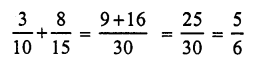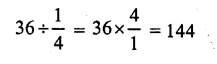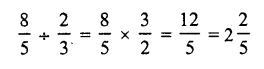# RS Aggarwal Solutions Class 7 Chapter 2 Fractions Ex 2D

In this chapter, we provide RS Aggarwal Solutions for Class 7 Chapter 2 Fractions Ex 2D for English medium students, Which will very helpful for every student in their exams. Students can download the latest RS Aggarwal Solutions for Class 7 Chapter 2 Fractions Ex 2D Maths pdf, free RS Aggarwal Solutions Class 7 Chapter 2 Fractions Ex 2D Maths book pdf download. Now you will get step by step solution to each question.

### RS Aggarwal Class 7 Solutions Chapter 2 Fractions Ex 2D Download PDF

OBJECTIVE QUESTIONS
Mark (√) against the correct answer in each of the following:
Question 1.
Solution:
(c)
Denominator in (a) and (b) is 10
These are decimal fractions
But denominator of (c) is 3
103 is a vulgar fraction

Question 2.
Solution:
(c)
710 and 79 are proper fractions as each of these have numerator less than its denominator
97 is improper fraction

Question 3.
Solution:
(a)
105112 is reducible fraction because HCF 112 of 105 and 112 is 7

Question 4.
Solution:
(c)Question 5.
Solution:
(c)Question 6.
Solution:
(d)Question 7.
Solution:
(c)Question 8.
Solution:
(d)Question 9.
Solution:
(d)Question 10.
Solution:
(b)Question 11.
Solution:
(d)Question 12.
Solution:
(c)Question 13.
Solution:
(d)Question 14.
Solution:
(d)Question 15.
Solution:
(b)
The correct statement will beQuestion 16.
Solution:
(c)
A car runs in 1 litre of petrol = 16 kmQuestion 17.
Solution:
(a)All Chapter RS Aggarwal Solutions For Class 7 Maths

—————————————————————————–

All Subject NCERT Exemplar Problems Solutions For Class 7

All Subject NCERT Solutions For Class 7

*************************************************

I think you got complete solutions for this chapter. If You have any queries regarding this chapter, please comment on the below section our subject teacher will answer you. We tried our best to give complete solutions so you got good marks in your exam.

If these solutions have helped you, you can also share rsaggarwalsolutions.in to your friends.